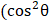# A force exerts an impulseon a particle changing its speed from initial velocityto final velocity 2The applied force and the initial velocity are oppositely oriented along the same line. The work done by the force is a)b)c)d)## Question ID - 100209 :- A force exerts an impulseon a particle changing its speed from initial velocityto final velocity 2The applied force and the initial velocity are oppositely oriented along the same line. The work done by the force is a)b)c)d)3537

(b)Next Question :

An elastic collision taken place between two smooth, rubber balls same radius as shown in fig. Initially, one ball is at rest and the other is moving with velocity. At maximum compressioni. ratio of potential to initial KE of the system is)/2

ii. ballis moving along directioniii. ballis moving along directionoriv. value ofcan not be used to finalise maximum compression velocities

Evaluate the above statements and choose the correct option from the following:

 a) Statements i, ii are true and iii, iv are false b) Statements  i, ii are false and iii,iv are true c) All statements are true d) All statements are false